maths > wholenumbers

Numerals 0 to 9

what you'll learn...

overview

•  count of real life objects

•  count of abstracted-blocks

The introduction with abstracted-blocks sets up learning the following concepts in the pages to come.

•  grouping to 10s, 100s, etc.

•  de-grouping in subtraction and division

•  regrouping in addition and multiplication

Do you know already? Do you?

The three lessons
numbers 1 to 10,
numbers 10 to 1000,
Expanded form of numbers
revise what students have learned in primary school or till 5th standard.

The objective of these lessons is to establish a pattern that helps to understand the foundation of numerical arithmetics. Like,
how carry over works in addition (regrouping)
how borrow works in subtraction (de-grouping)
how large numbers are added, subtracted, multiplied, or divided (procedural simplification in place-value system)

These lessons take hardly $20$$20$ mins. Learners are advised to go through them noting how numbers are represented with units blocks, tens blocks, hundreds blocks, etc. . This helps in understanding explanation of various concepts in numerical arithmetics.

Digits, Numbers, Numerals

Children use fingers to count when they first start learning counting.

Another word for fingers is digits.

The numbers 0 to 9, which are used to count, are also called digits.

Numbers are used to represent counts or measures.

Number of pens is $5$$5$

Length of a pencil is $3$$3$cm

Numbers are represented using numerals. Hindu Arabic numerals use digits

$0,1,2,3,4,5,6,7,8,9$$0 , 1 , 2 , 3 , 4 , 5 , 6 , 7 , 8 , 9$

Digits : $0$$0$, $1$$1$, $2$$2$, $3$$3$, $4$$4$, $5$$5$, $6$$6$, $7$$7$, $8$$8$, $9$$9$ are called digits.

Number : A number is a count or a measurement of a quantity.

Numeral : A numeral is a number represented with digits.

Count and Measure

Let us quickly revise the numbers. This brings out the foundation of numbers and its place value system. Since it is very easy, just spend time going through once.

This part is designed such that the foundation of arithmetics is understood in the right way.

For example, in this page, the representation of numbers as count and measure is explained and a representation that reflects the place-value system of numbers is developed which helps in understanding arithmetics.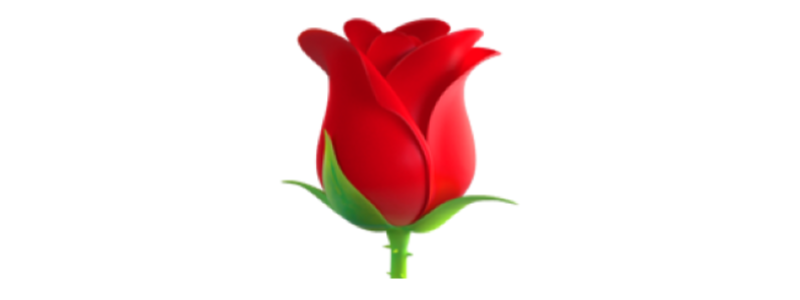The number of dogs in the picture is $0$$0$.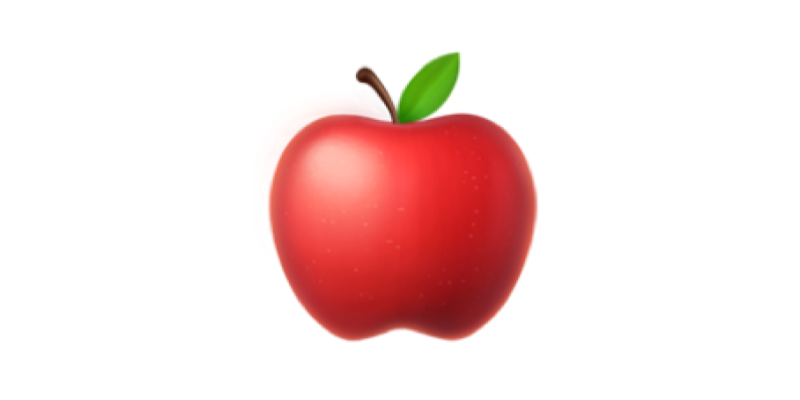The number of apples in the picture is $1$$1$.The number of blocks in the picture is $1$$1$.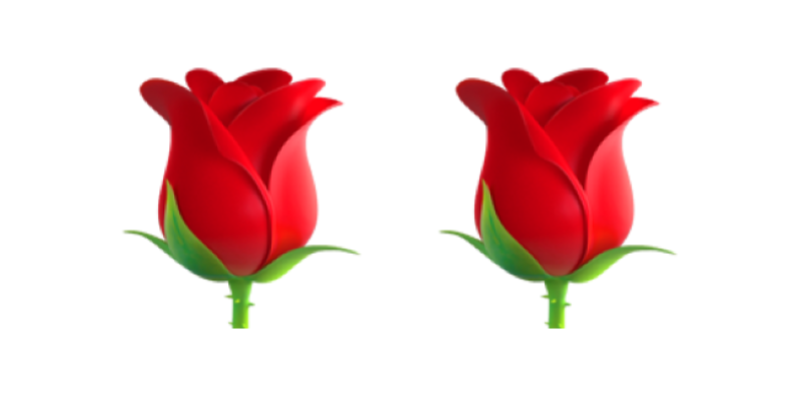The number of roses in the picture is $2$$2$.The number of blocks in the picture is $2$$2$.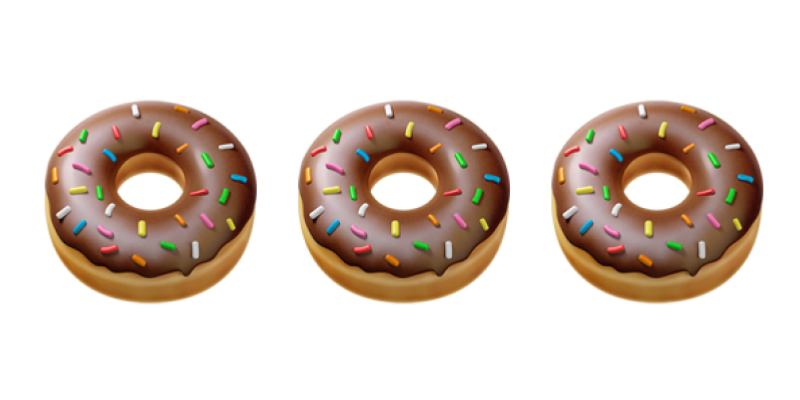The number of donuts in the picture is $3$$3$.The number of blocks in the picture is $3$$3$.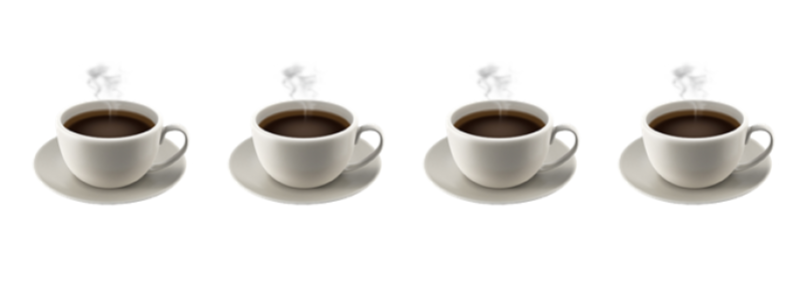The number of cups in the picture is $4$$4$.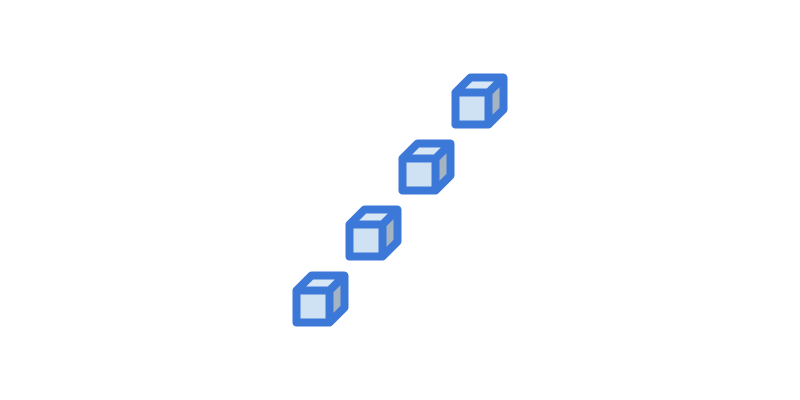The number of blocks in the picture is $4$$4$.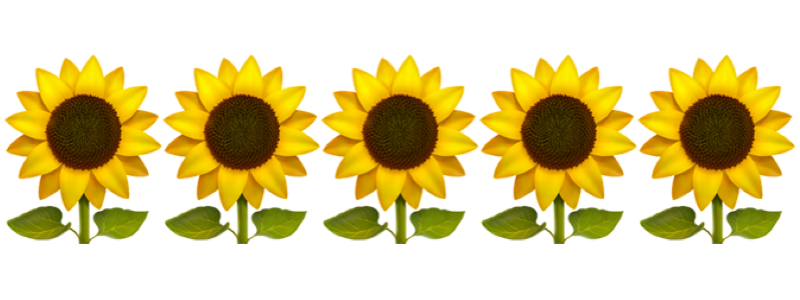The number of flowers in the picture is $5$$5$.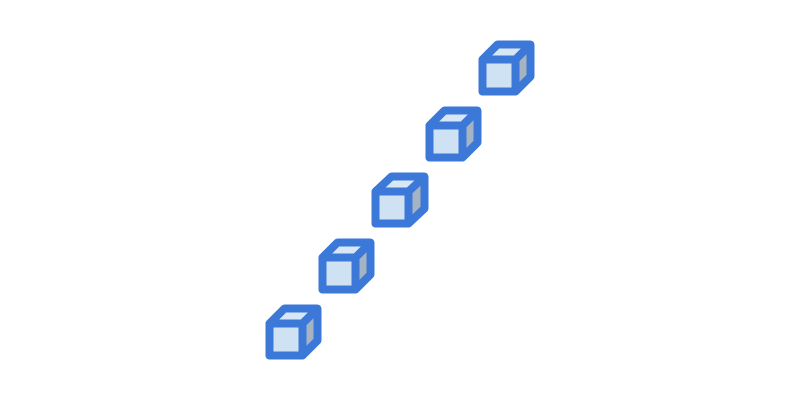The number of blocks in the picture is $5$$5$.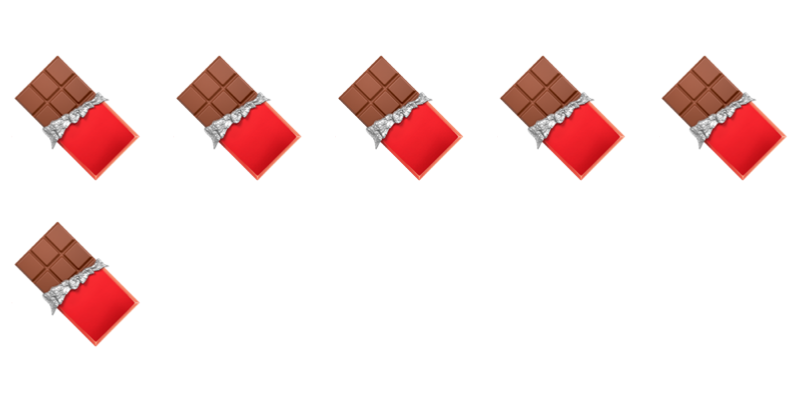The number of chocolates in the picture is $6$$6$.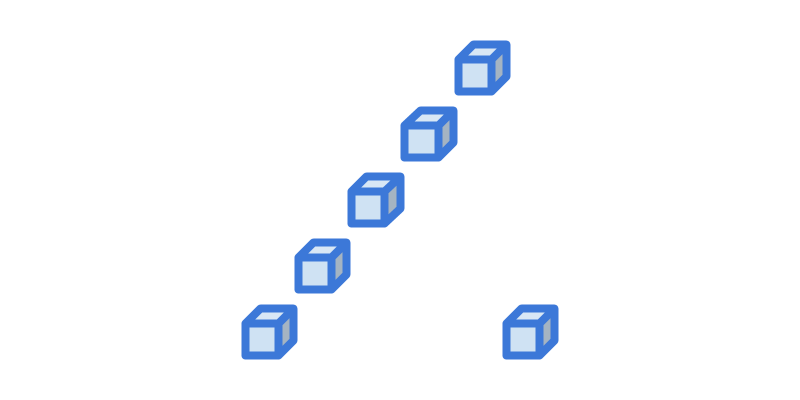The number of blocks in the picture is $6$$6$.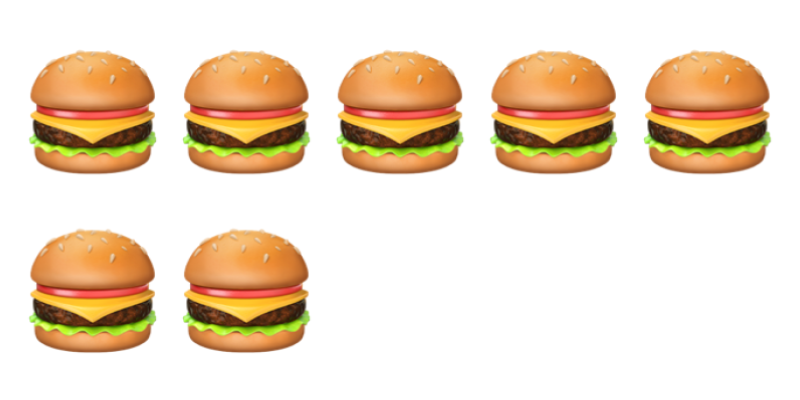The number of burgers in the picture is $7$$7$.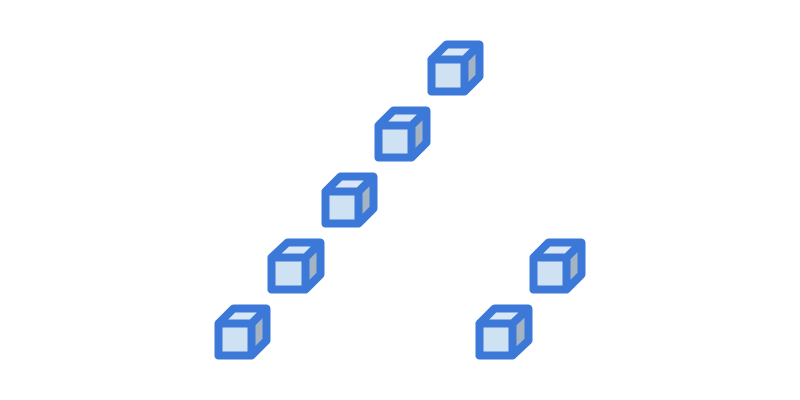The number of blocks in the picture is $7$$7$.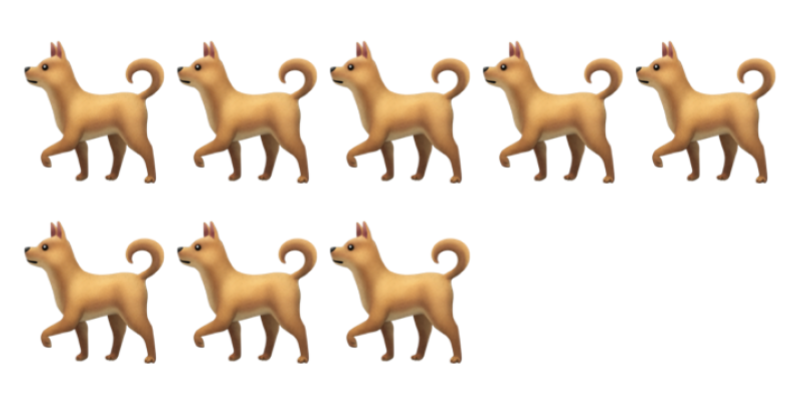The number of dogs in the picture is $8$$8$.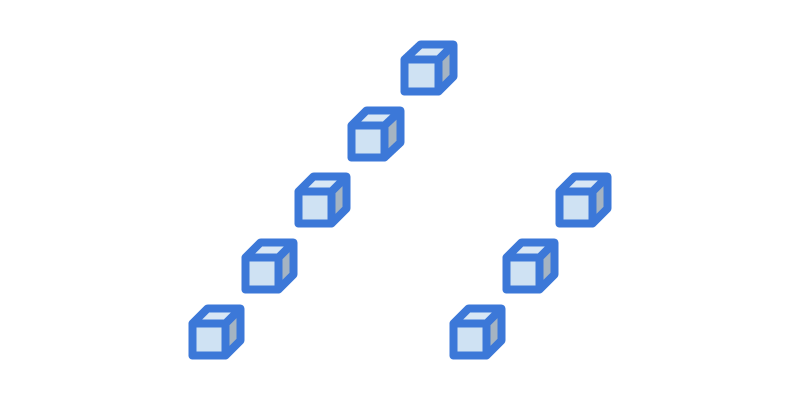The number of blocks in the picture is $8$$8$.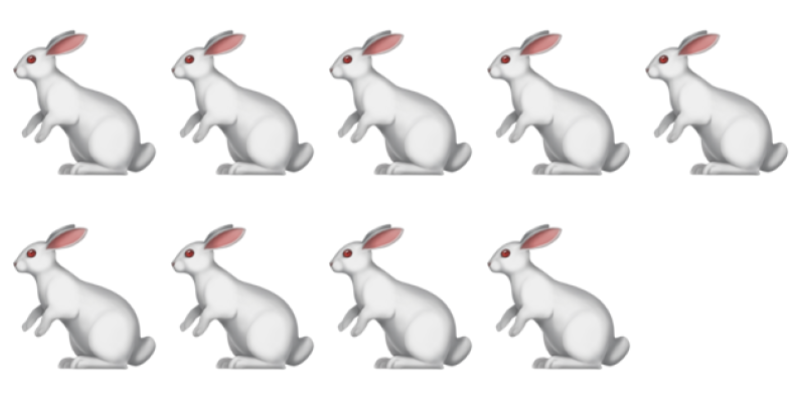The number of rabbits in the picture is $9$$9$.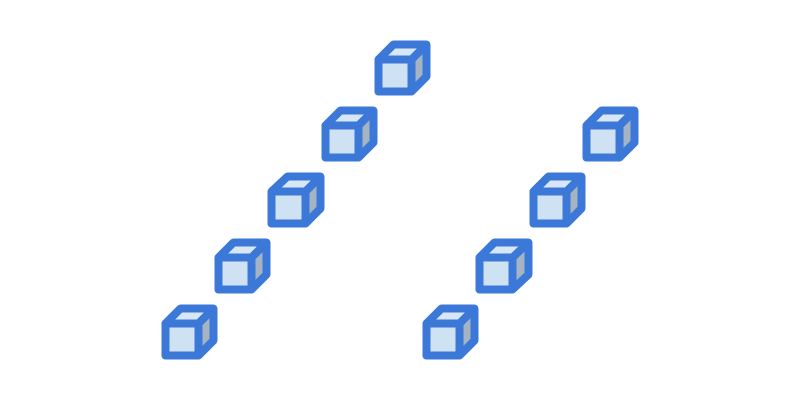The number of blocks in the picture is $9$$9$.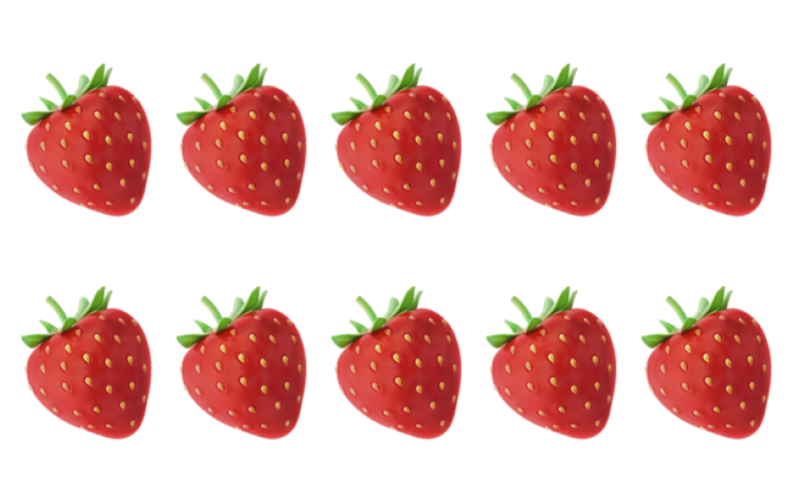The number of strawberries in the picture is $10$$10$.The number of blue blocks in the picture is $10$$10$.

These blue blocks are combined into a single purple block.

Example :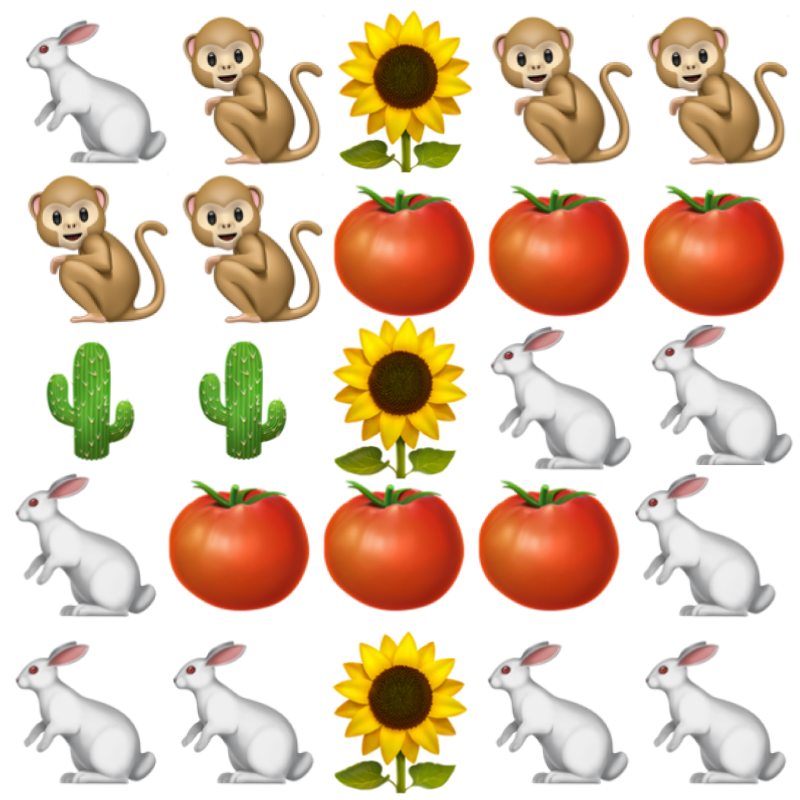The number of flowers in the picture is $3$$3$.

The number of cacti is $2$$2$.

The number of monkeys is $5$$5$.

The number of tomatoes is $6$$6$.

The number of rabbits is $9$$9$.

Summary

Numbers $1,2,3,4,5,6,7,8,9,10$$1 , 2 , 3 , 4 , 5 , 6 , 7 , 8 , 9 , 10$ and $0$$0$ are introduced as count of objects.

The numbers corresponding to real world objects can also be represented using abstracted blocks.The number of donuts in the picture is $3$$3$.The number of blocks in the picture is $3$$3$.

Numbers: The numbers we use to count or measure quantities are

$0$$0$ (zero),
$1$$1$ (one),
$2$$2$ (two),
$3$$3$ (three),
$4$$4$ (four),
$5$$5$ (five),
$6$$6$ (six),
$7$$7$ (seven),
$8$$8$ (eight),
$9$$9$ (nine)

Outline